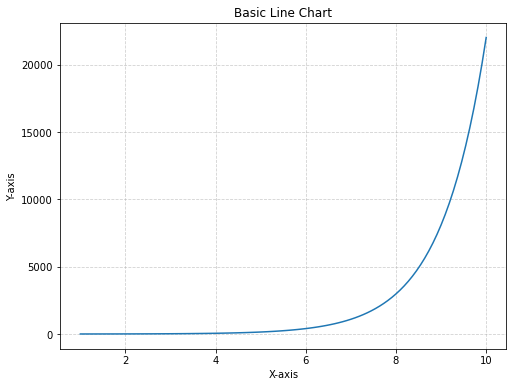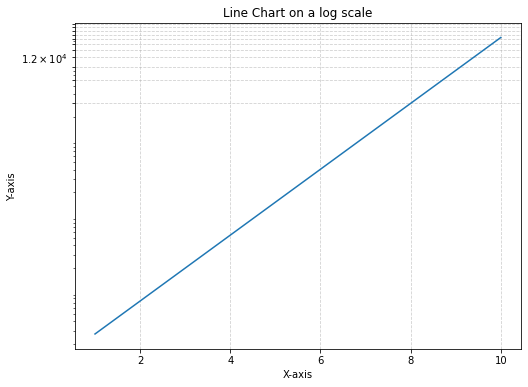### Principles

A line chart is a type of visual representation that uses a series of points connected by lines to show how data changes over time. It's like connecting the dots on a graph to reveal trends and patterns. Line charts are useful for tracking things that happen over a period, such as temperature changes, stock prices, or your savings in a piggy bank. They help us quickly see if something goes up, down, or stays steady as time passes, making it easier to understand how things are changing and make informed decisions.

### Log transform

In some cases, the data you're working with might span a wide range of values, making it difficult to visualize on a traditional linear scale. This is where a `log transformation` can come in handy. A log transformation involves taking the logarithm of the data values, which can help compress the range of values and reveal patterns that might be otherwise obscured. This is particularly useful when you have data with exponential growth or decay, as the `log transformation` can linearize such relationships.

## Libraries

First, you need to install the following librairies:

• matplotlib is used for plot creating the charts (and the `MultipleLocator` for showing the log scale)
• `numpy` is used to generate some data
• `pandas` is used to put the data into a dataframe
``````# Libraries
import matplotlib.pyplot as plt
from matplotlib.ticker import MultipleLocator
import numpy as np
import pandas as pd``````

## Dataset

Line chart are generally used to represent variations over time, but they don't have to be. For example, you can take a look at connected scatter plots.

In our case, we will generate values using `np.linespace()` and `np.exp()` functions from `numpy`.

``````# Generate a variable
x = np.linspace(1, 10, 100)  # Create 100 points from 1 to 10
y = np.exp(x)                # Exponential growth

# Create the dataframe
df = pd.DataFrame({'x': x,
'y': y})``````

## Line chart without log transformation

The following code displays a simple line chart, with a title and an axis name, using the `plot()` function. We don't apply a logarithmic transformation, which makes the graph difficult to read.

For example, we are unable to say whether or not the y-axis has increased in the first (more or less) 14 values.

``````# Create a figure and axis
fig, ax = plt.subplots(figsize=(8, 6))

# Create the line chart using the axis
ax.plot(df['x'],
df['y'])

# Add labels and a title
ax.set_xlabel('X-axis')
ax.set_ylabel('Y-axis')
ax.set_title('Basic Line Chart')

ax.grid(True, linestyle='--', alpha=0.6)

# Display the chart
plt.show()``````## Line chart with log transformation

With matplotlib, it's easy enough to set up a logarithmic scale: just add `ax.set_yscale('log')` to our code. The grid in the background is added using the `MultipleLocator()` function from matplotlib, where we add one line every 3000 steps.

Now we can clearly see that our y value is clearly increasing exponentially with our x value, thanks to our `log scale`!

``````# Create a figure and axis
fig, ax = plt.subplots(figsize=(8, 6))

# Create the line chart using the axis
ax.plot(df['x'],
df['y'])

# Set the y-axis to a log scale
ax.set_yscale('log')

# Add labels and a title
ax.set_xlabel('X-axis')
ax.set_ylabel('Y-axis')
ax.set_title('Line Chart on a log scale')

# Add the grid for the log scale
y_major_locator = MultipleLocator(3000)  # Set the grid interval to 3000
ax.yaxis.set_major_locator(y_major_locator)

# Add a grid with a log scale
ax.grid(True, linestyle='--', alpha=0.6)

# Display the chart
plt.show()``````## Going further

This article explains how to create a line chart with logarithmic scale with Matplotlib.

For more examples of how to create or customize your line charts with Python, see the line chart section. You may also be interested in creating a line chart with multiple groups to your chart.

## Contact & Edit

👋 This document is a work by Yan Holtz. You can contribute on github, send me a feedback on twitter or subscribe to the newsletter to know when new examples are published! 🔥